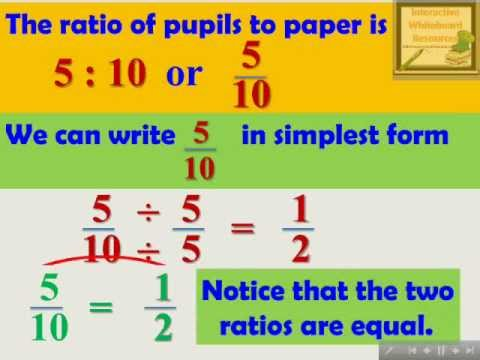## Ratio and Proportion

Subject: Compulsory Maths

#### Overview

The ratio shows the relative sizes of two or more values. If there is the equality between two ratios then it can be known as the proportion. If the ratio of the number of articles and their cost is equal then it is called direct proportion. If the ratio of the number of workers and the ratio of their working days are exactly opposite then it is called indirect proportion.
##### Ratio and Proportion

Ratio

The ratio shows the relative sizes of two or more values. The ratio compares two or more quantities of the same unit. It tells how many times quantity is less or more than another quantity of the same unit.

For example, The ratio of a to b = a:b or $\frac{a}{b}$. Similarly ratio of b to a = b:a or$\frac{b}{a}$. The quantities of a ratio are called the terms of the ratio. The term in the numerator is called antecedent and the denominator is called consequent.

Proportion

If there is the equality between two ratios then it can be known as the proportion. Let a, b, c and d are called first, second, third and fourth proportional respectively, The first and fourth proportional are called Extremes. The second and third proportional are called Means.Types of proportion

1. Direct Proportionsource: www.math-for-all-grades.comFig: Direct Proportion If the ratio of the number of articles and their cost is equal then it is called direct proportion. If the number of articles increases or decreases and their cost also increases or decreases. For example,
The cost of 1 articles = Rs.15
Then, the cost of 5 articles = 5× Rs.15 = Rs.75
∴ Ratio of the number of articles = 1:5
The ratio of cost of pens = 15:75 = 1:5
2. Indirect Proportionsource: slideplayer.com Fig: Indirect Proportion If the ratio of the number of workers and the ratio of their working days are exactly opposite then it is called indirect proportion. For examples,
1 man can finish a piece of work in 20 days. Then 2 men can finish the same work in 5 days.
Here the ratio of the number of men = 1:4
the ratio of the working days = 20:5 = 4:1

##### Things to remember
• The ratio tells how many times quantity is less or more than another quantity of the same unit.
•  The term in the numerator is called antecedent and the denominator is called consequent.
• The first and fourth proportional are called Extremes.
• The second and third proportional are called Means.
• It includes every relationship which established among the people.
• There can be more than one community in a society. Community smaller than society.
• It is a network of social relationships which cannot see or touched.
• common interests and common objectives are not necessary for society.
##### Videos for Ratio and Proportion##### Ratio and Proportion - Interactive Mathematics Lesson Module

Soln:

Here, 1m=100 cm

Ratio of 100 cm and 75 cm = 100 :75

=$\frac{100}{75}$

=$\frac{4}{3}$ =4:3

Solution :

let, the consequent be x.

Now , $\frac{25}{x}$ = $\frac{5}{7}$

or , 5x =25 x 7

or , x = $\frac{25\times7}{5}$

or, x = 35 .

So, the required consequent is 35.

Solution :

Let , the age of mother be x year.

$\frac{x}{24}$ =$\frac{9}{4}$

or, 4x =24 x 9

or, x = $\frac{24 × 9}{4}$=54

So , the mother is 54 years old .

Solution :

Let the fourth proportional be x.

Now , if 2,3,14 and x are in proportion .2:3= 14: x

or , $\frac{2}{3}$ = $\frac{14}{x}$

or , 2x = 3 x14

or x = $\frac{3\times14}{2}$ = 21

So , the required fourth proportional is 21.

Solution :

the product of extremes = 3 x 16 =48

the product of means = 4 x 12 =48

$\therefore$ Product of extremes = Product of means

$\therefore$ 3:4=12:16

So, 3:4 and 12:16 are in propertion .

Solution:

Let, the required cost be Rs x.

Here, the quantities of apples and their cost are in direct proportion.

$\therefore$ 8:5 = 180:x

or, $\frac{8}{5}$ = $\frac{280}{x}$

8x = 5 × Rs 280

x = $\frac{5 ×Rs 280}{8}$ = Rs 175.

So the required cost is Rs 175.

Solution:

Here, Rs 2 is the tax for the income of Rs 100

Re 1 is the tax for the income of Rs $\frac{100}{2}$ = Rs 50

Rs 525 is the tax for the income of Rs 525 × 50 = 26250.

So, the required income of the man is Rs 26250.

Solution:

In 8 days, Hari can do 1 work.

In 1 day, Hari can do $\frac{1}{8}$ part of the work.

In 6 days, Hari can do $\frac{6}{8}$ parts of work = $\frac{3}{4}$ parts of the work.

Now, remaining parts of the work = (1 - $\frac{3}{4}$) = $\frac{1}{4}$ part of the work.

So, Gopal finished $\frac{6}{8}$ parts of the work.